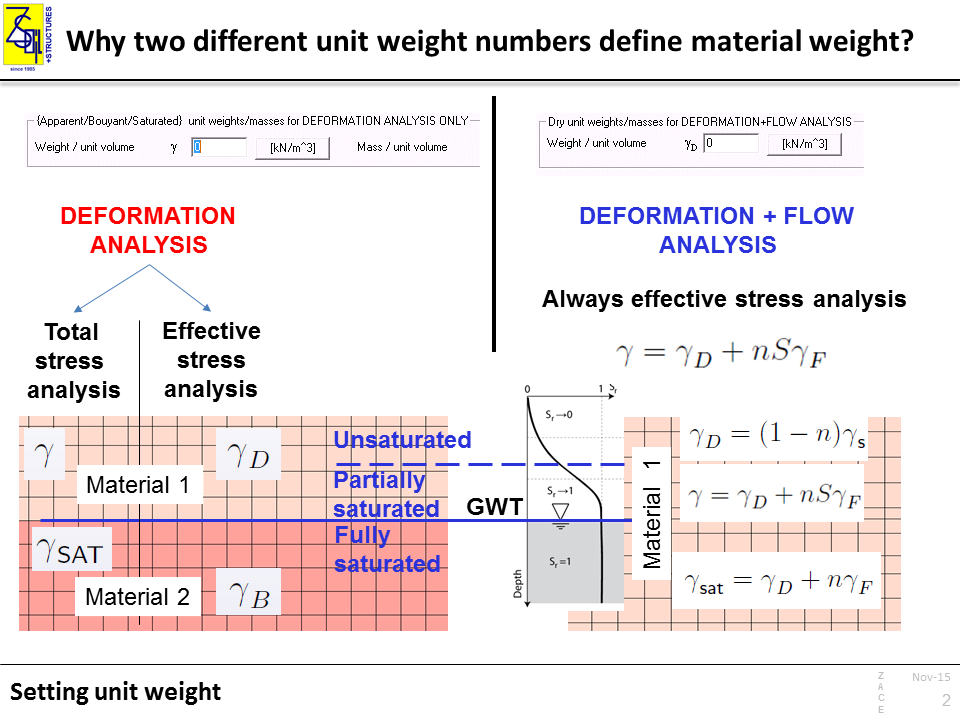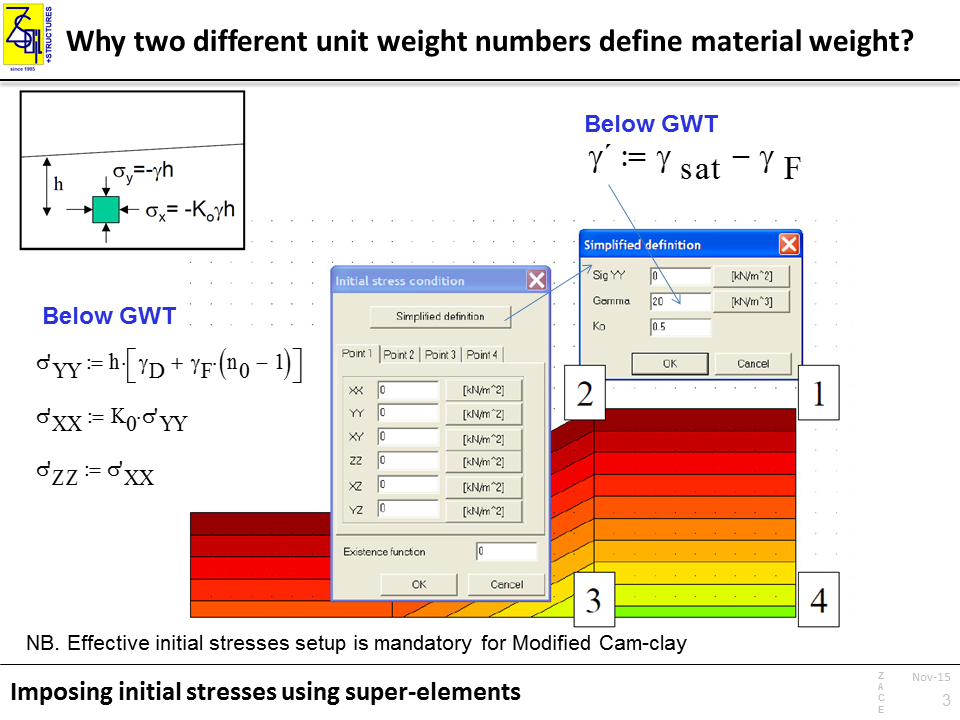# Why two different unit weight numbers define material weight?

Two different unit weights define material weight for two different types of numerical analysis:

1. Deformation analysis for which material is considered as a single-phase medium

2. Deformation + Flow analysis where material is a double-phase medium which consists of skeleton and fluid that fills voids

The Deformation + Flow analysis is always considered as the effective stress analysis. In this case, material consists of skeleton and voids which can be filled with water.

Therefore, an apparent weight of each finite element depends on unit weight of dry material gD, its current porosity n, and the saturation degree S. It means that the weight may vary for the same material depending on saturation conditions.

In the case of Deformation analysis (single-phase continuum), material’s weight remains constant throughout the entire simulation. If the material is considered as a solid-fluid mixture, one has to decide which type of analysis is intended to be carried out:

1 . Total stress analysis – used when modelling undrained behavior using total stress parameters, e.g. φ = 0, c = Su (undrained shear strength)

or

2. Effective stress analysis – using effective stress parameters and assuming free drainage conditions in the real conditions.

In both cases, a ground water table (GWT), if such exists, should be taken into account. Therefore, geotechnical layer should be discretized in the model with the aid of two different materials although they share the same mechanical parameters.

The following unit weights can be assumed:

1.Total stress analysis – unit weight of soil with natural moisture γ above GWT, and unit weight of saturated soil γSAT below GWT

2.Effective stress analysis – unit weight of dry soil, γD, should be given to compute the profile of effective stresses above GWT, whereas buoyant weight of soil γB = γSAT – γF should be defined for the fully saturated soilIn some cases the initial stress can be imposed by the user be means of so-called super-elements.

Imposing initial stresses can solve convergence issues in the case of the boundary value problems that include construction works, e.g. an embankment. The initial stresses should be then imposed on the introduced finite elements. Imposing initial stresses is mandatory for the Modified Cam-clay model since the initial shear modulus is computed based on the mean effective stress.

Initial stresses super-elements can be defined in PreProcessor (Initial Conditions -> Initial Stresses), by selecting 4 nodes (2D) or 8 nodes (3D) . Note that the compressive stresses are negative numbers. In the case of Deformation+Flow analysis the imposed initial stresses always define effective stresses.